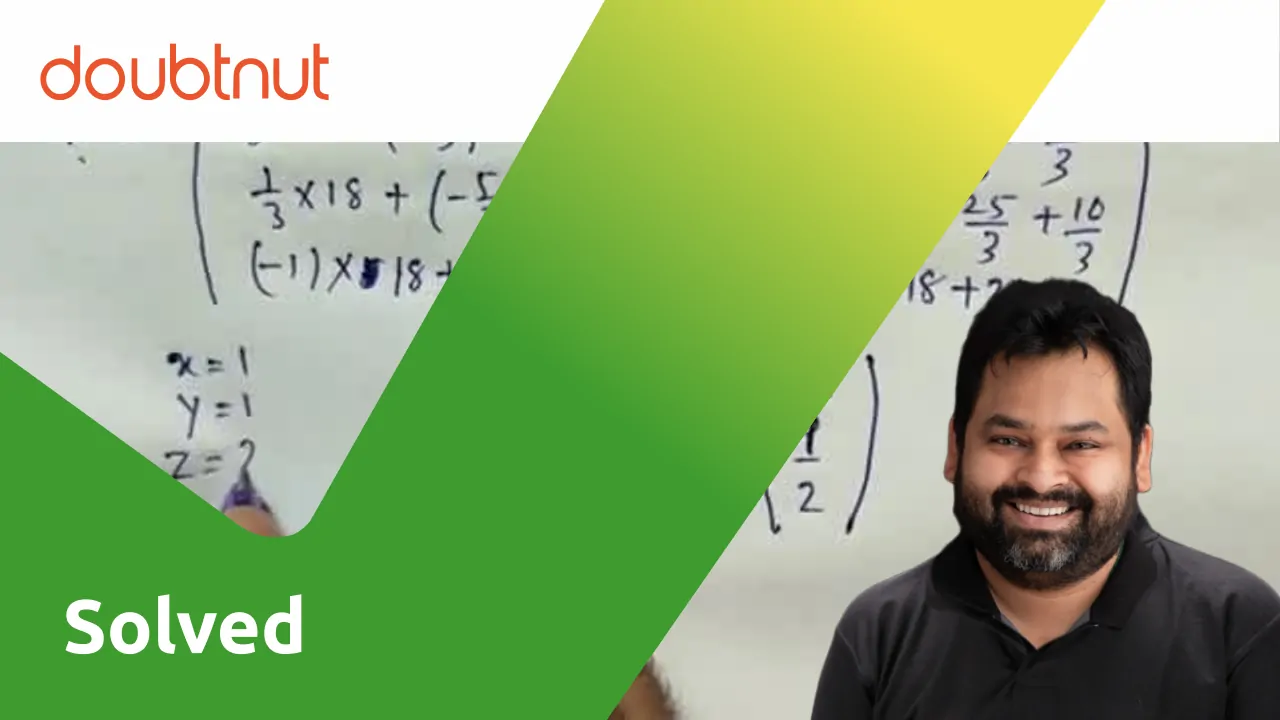Doubtnut App - Get 50 Lakh+ video solutions better than GoogleInstall Now

# Solve by matrix inversion method the following system of equations. 8x + 4y + 3z = 18, 2x + y + z = 5, x + 2y + z = 5 Solution in Bengali

Text Solution
Verified by Experts

## The correct Answer is:x =1, y = 1, z = 2

Video Solution